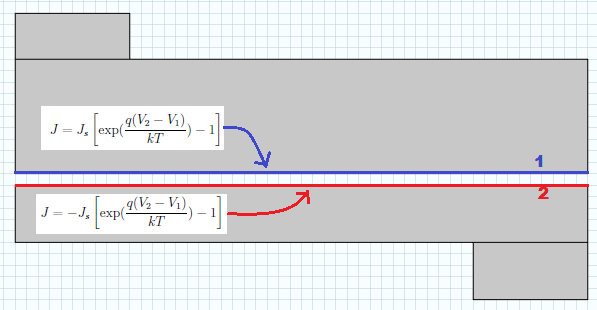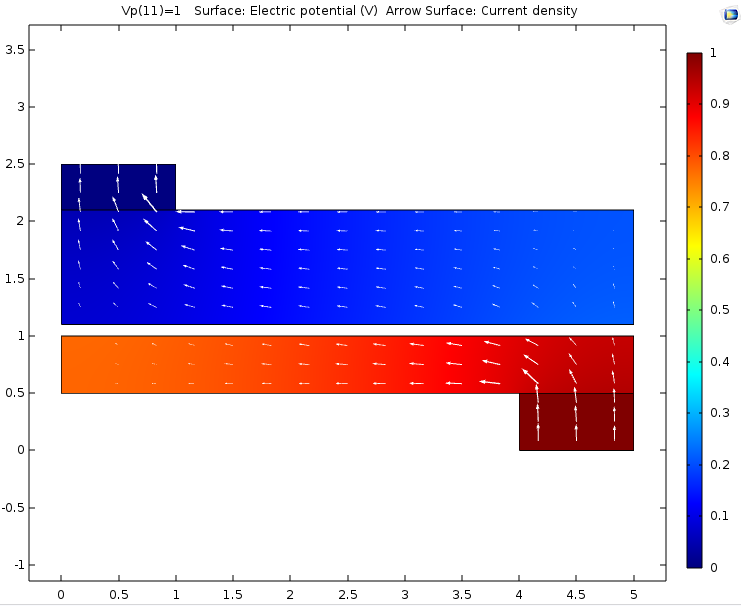# 第 2 部分：用广义拉伸算子映射变量

2015年 10月 5日

### 拉伸算子简要回顾

\textbf{T}:P_d \rightarrow P_s.

### 在 COMSOL Multiphysics 中使用广义拉伸算子

#### 示例1

x_s = ax_d + by_d + e, \qquad y_s = cx_d + dy_d + f.

x_s = \frac{2}{3}x_d,
y_s = \frac{2}{3}y_d.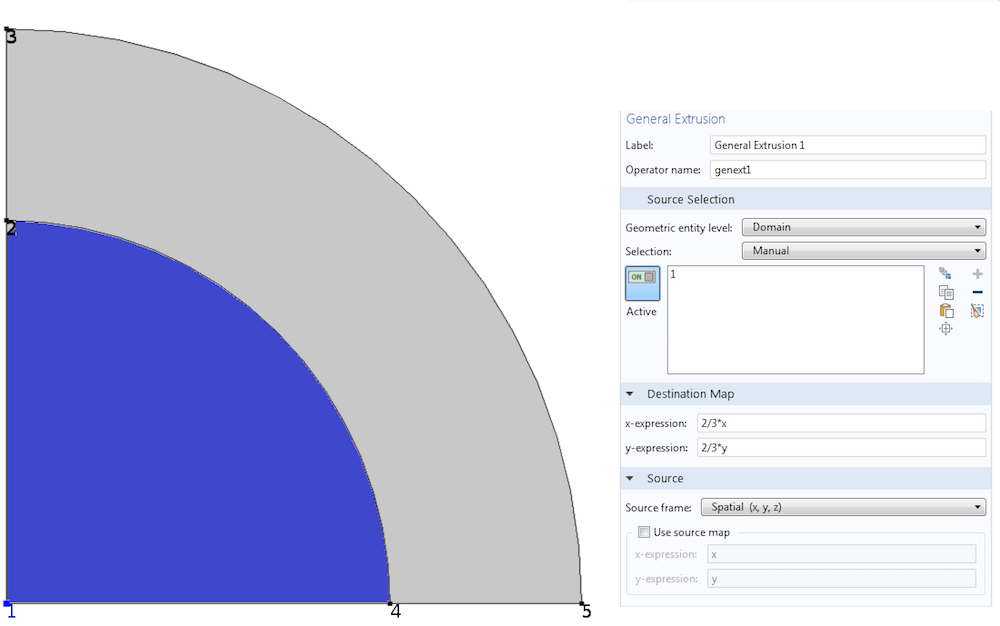#### 示例 2

r_s = \sqrt{x_d^2 + y_d^2}, \qquad z_s = z_d.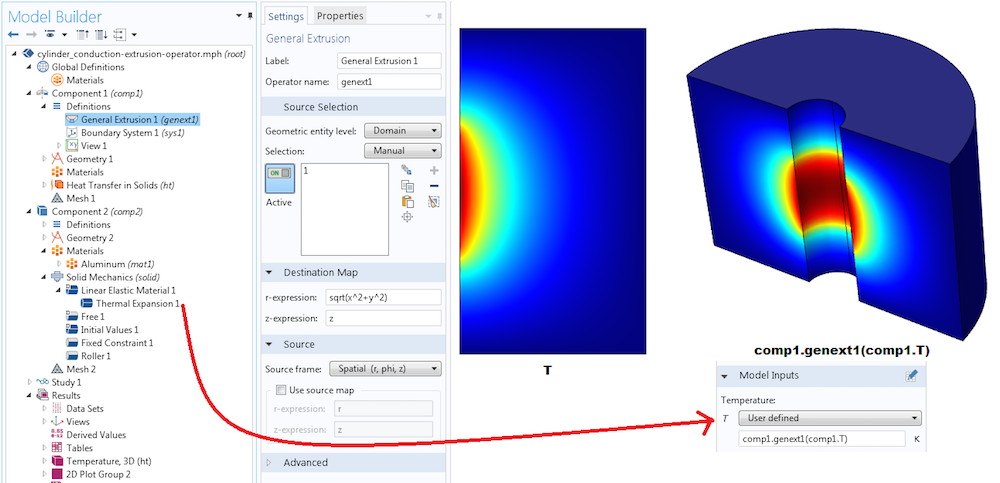#### 示例 3

2D 抛物线由 \frac{y}{d} =(\frac{ x}{d})^2 给出，它位于边 d 的正方形域内。我们的任务是构建一个算子，将这条曲线(下图中用蓝色表示)的数据映射到正方形的不同部分。抛物线是源。我们想要从抛物线上的一个点复制到正方形中的一个点的算子，例如目标点到原点的距离等于原点和源点之间的抛物线段的长度。

L(x,y) = \int_{o}^{x}\sqrt{1+(y\prime)^2}d\tau = \frac{x}{2}\sqrt{1+4(\frac{x}{d})^2}+\frac{d}{4}\ln(2\frac{x}{d}+\sqrt{1+4(\frac{x}{d})^2}).

\frac{x_s}{2}\sqrt{1+4(\frac{x_s}{d})^2}+\frac{d}{4}\ln(2\frac{x_s}{d}+\sqrt{1+4(\frac{x_s}{d})^2}) = \sqrt{x_d^2+y_d^2}.

x_s = T(x_d,y_d),

T_s(x_s,y_s) = T_d(x_d,y_d).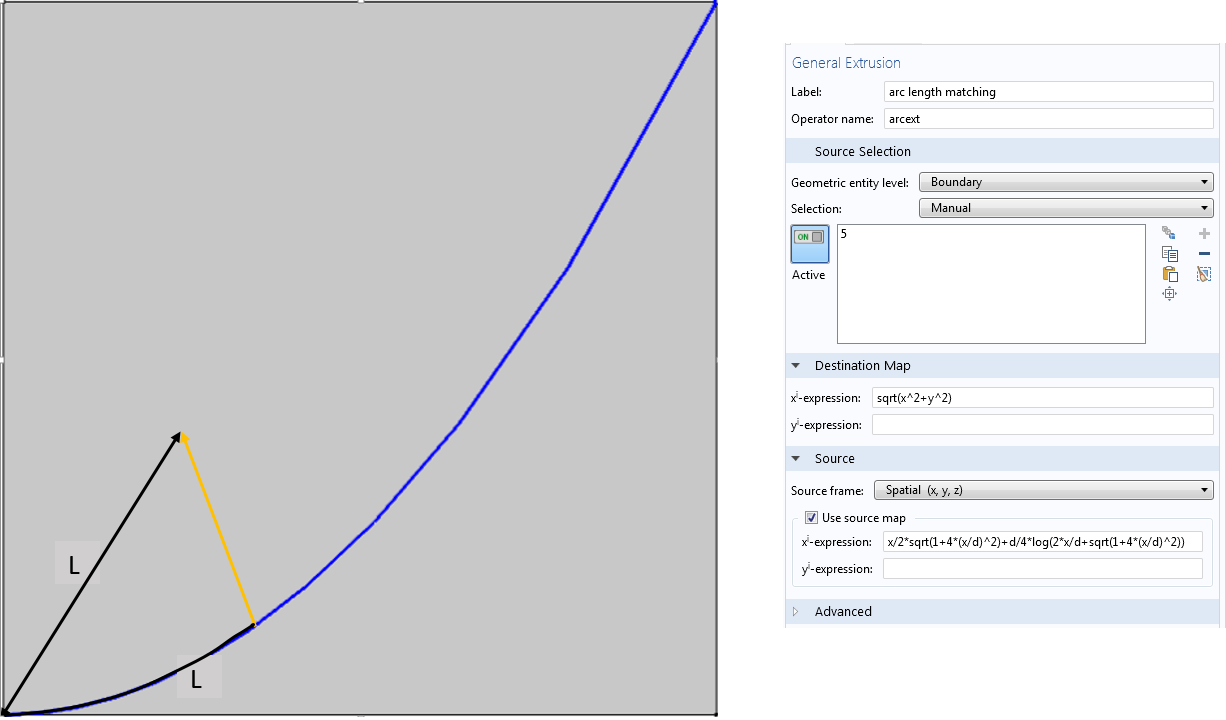COMSOL Multiphysics 会处理 T_s^{-1}(T_d(x_d,y_d))，这是识别源坐标的必要步骤。请注意，源映射需要一对一，这样逆映射才能存在。实际上，COMSOL Multiphysics 并不为源映射逆构造解析表达式。相反，在每个目标点，它首先计算 T_d(x_d,y_d) 并且在源上执行网格搜索操作以找到源上匹配的点 T_s(x_s,y_s)。一对一的源映射使搜索最多返回给定目标点一个源点。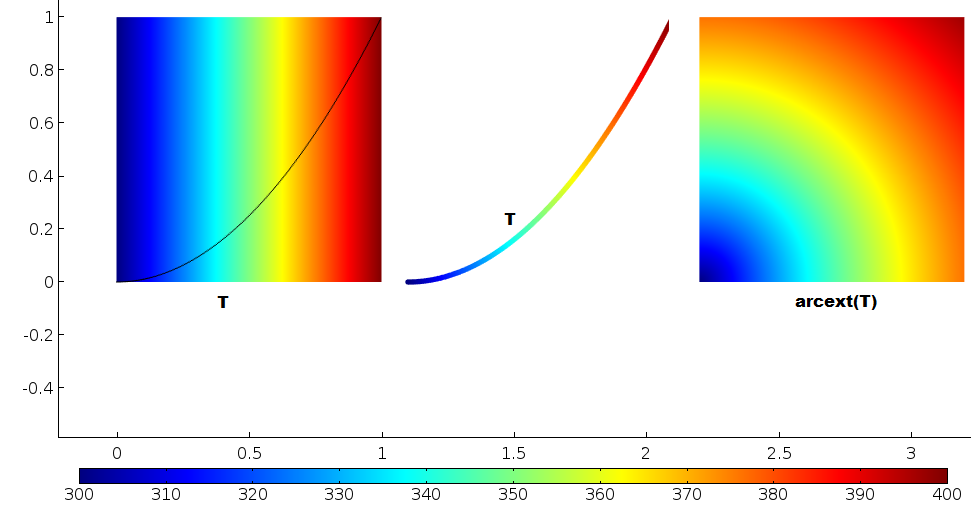### 将一切拼凑在一起